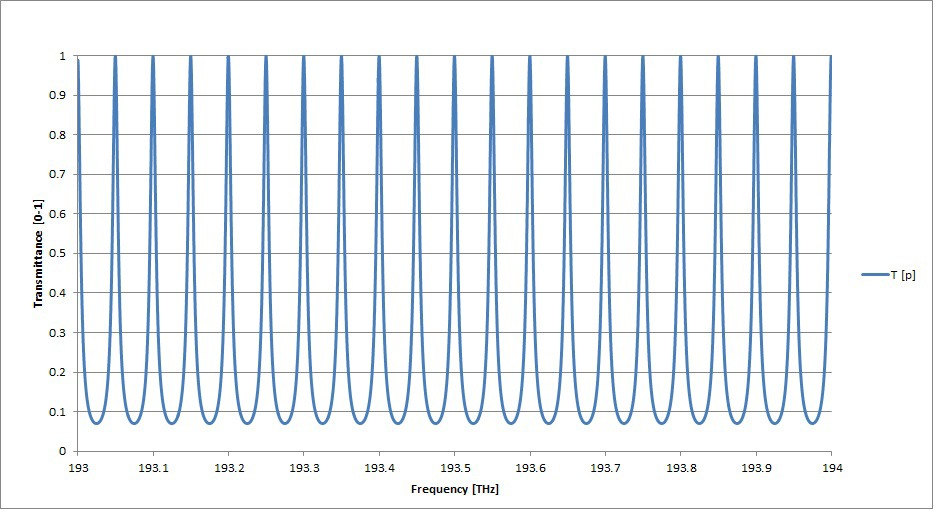# Etalon TutorialA Solid Etalon Filter (SEF) is used to select a periodically spaced frequency grid from a broad spectral range. The key specifications of these filters are Free Spectral Range (FSR), and Finesse. The FSR of a SEF is equal to the spacing between the transmittance peak frequencies. The finesse is said to describe the sharpness of the transmittance peaks and is equal to the FSR divided by the full width at half maximum (FWHM) of the transmittance peak frequencies.

The SEF is a version of a simple etalon, Mirror|Spacer|Mirror, in which thin film dielectrics are used as the mirrors and a solid glass substrate is used as the spacer. Iridian’s SEFs are made up of two plane parallel reflecting surfaces, side A & B, separated by a quartz substrate with thickness “d”.

The SEF is specified/quantified primarily by two parameters: Free Spectral Range (FSR) & Finesse.

## Free Spectral Range (FSR) [GHz]

The Free Spectral Range (FSR) is the separation between two adjacent peak frequencies fi and fi+1:

FSR = fi – fi+1

Neglecting the effect of dispersion in the quartz spacer, the FSR can be predicted by the following equation:

FSR = c/( 2·n·d·cos(Ø) )

where c is the speed of light, n is the refractive index of the spacer, d is the quartz substrate thickness, and Ø is the angle of incidence. For Ø=0 and constant refractive index, the FSR of a SEF is inversely proportional to the spacer thickness:

FSR ~ d-1

Then small changes in FSR can be related to small changes in spacer thickness:

D(FSR)/FSR = -D(d)/d

## Finesse (F)

The finesse (F) characterizes the sharpness of the peak frequencies of a SEF:

F=FSR/(-3 dB BW)

where -3 dB BW is the bandwidth at –3.0 dB (or the full width at half maximum) of the peak frequencies.

The finesse is also a function of the reflectance, R, of the reflecting surfaces A & B:

F = pR1/2/(1-R)